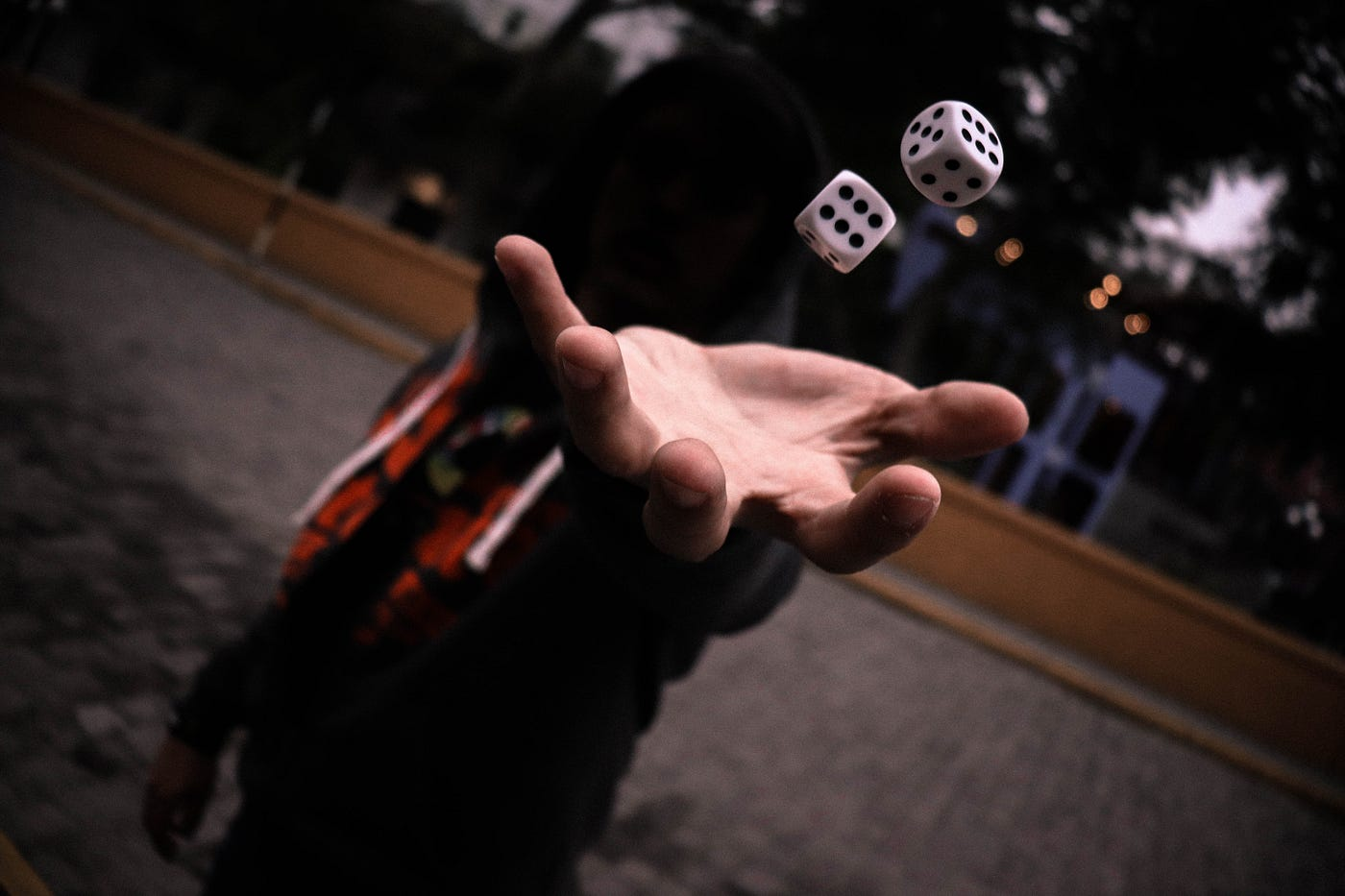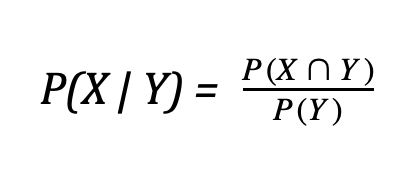# An Introduction to Probability and the World of Chance

## Exploring the meaning and use of probabilityPhoto by Guillermo Velarde on Unsplash

The concept of probability touches practically all aspects from our lives. In fact, we base many of the decisions we make on the probability of events we perceive to be the most beneficial. Therefore, it is crucial to obtain a decent understanding of probability theory and learn how to apply it to other problems.

Usually, the first scenarios that come to mind when we think about probability are dice or cards. After all, both of these objects are often associated with games, gambling, or anything with chance involved. Interestingly enough, the term “chance” actually quite accurately sums up the definition of probability. Put in simple terms, probability is just how likely something is to occur.

“It is a part of probability that many improbable things will happen.”

-Aristotle

However, if we want to get a little more mathematical, we can describe the idea of probability as a fraction.

P(E) = (the number of ways E is true) / (the total number of outcomes)

where P(E) represents the probability of an event occuring.

The value of P(E) will always be a decimal value in the interval [0 , 1] (though this decimal can be multiplied by 100 to obtain a percent); the higher the value, the more likely the event will occur, and a probability of 0 indicates the impossibility of an event occurring, while a probability of 1 indicates irrevocable certainty. Now, let’s look at a few simple yet informative examples of how our definition works in the context of a problem.

What is the chance of rolling a three on a normal dice?

This question can be solved relatively quickly using intuition, but let’s try to apply the previously generated formula. There is only 1 side that displays the number three on it, and there are 6 sides in total. By applying the definition, we can now clearly see that P(“three”) = 1 / 6.

What is the chance of picking a Jack from a normal pile of playing cards?

Again, we need to ask ourselves the essential questions: How many ways can we choose a jack? How many total cards are we selecting from? Well, we know that a standard deck of playing cards contains 52 cards, with 4 face cards each, meaning a total of 4 jacks. Therefore, the probability of picking a jack is 4 / 52 or 1 /13.

Both of the previous two examples are relatively simple questions that are meant to guide a person with minimal experience into the field of probability. The reality of the situation is that there are many more complicated mathematical methods of analysis needed to handle some of the more difficult problems.

# Probability of Unions and Intersections

A union is one of the two common types of compound events (when there are two or more events involved). We define the probability of the union of events X and Y to be the probability that either X or Y or both occur. From this definition, two different formulas arrive for calculating the probability depending on if events X and Y can occur simultaneously or not: it depends on their mutual exclusivity.

If two events can not occur at the same time, they are deemed mutually exclusive, whereas if they can occur at the same time, they are deemed not mutually exclusive.

In the case that events X and Y are indeed mutually exclusive, we have the following equation.

For example, let’s say we wanted to calculate the probability of rolling a five or a six. These events can not occur at the same time, so we just need to add up the individual probabilities. The chance of rolling a five is 1 /6, and the chance of rolling a six is also 1 /6; therefore, the chance of rolling a five or a six is 1 / 3.

However, in the case that X and Y are not mutually exclusive, the formula becomes slightly altered.

As you’ll notice, there is an extra probability attached to the end of this equation with a symbol that sort of resembles an upside-down U. This is called the intersection, the second of the two common types of compound events. The probability of the intersection of two events is defined as the probability of both event one and event two occurring in one run. We will discuss the intersection formula, but the fact is that the concept of intersection is extremely important when dealing with probabilities of non mutually exclusive events.

A classic example that showcases the previous union formula is with cards. Suppose we want to determine the probability of drawing a spade or a queen. With cards, we now have to shift to the non mutually exclusive world, since it is possible to draw a card that is both a spade and a queen. According to our formula, we need to find the probability of selecting a spade, a queen, and the queen of spades, which is 13 / 52, 4 / 52, and 1 / 52, respectively. The final probability can be obtained by adding the first two fractions and then subtracting that sum by the last fraction. Doing so gives us 16 / 52 or 4 / 13.

Now that we’ve already been exposed to the concept of intersection, let’s delve a litter deeper and explore the formula. We typically deal with intersection in the context of independence, which is when the probability of one event does not affect the probability of the other. In this case, the formula for intersection is as follows.

For example, if two quarters are flipped, the chance that all they are both tails is 0.5 * 0.5 = 0.25. An alternative way to think about this problem is to look at our first definition of probability involving the number of times the event occurs and the total number of outcomes. First, let’s list all of possible outcomes of flipping two quarters.

{H,H} , {H, T} , {T , H} , {T ,T}

How many of the outcomes contain both tails? Only one out of four.

# Conditional Probability

Conditional probability refers to the probability of an event X given the occurrence of an event Y.Conditional probability of X, given Y

(You’ll notice that the expression for intersection is in this equation. If we isolate that term, we can see that it is equal to P(X | Y) * P(Y). This version of the intersection formula is used for events that are not independent of each other)

Suppose we are given some data about upperclassmen practice attendance in a certain week. The manager of the team created a chart with the following information about the 41 total students in question.

Out of the 22 juniors on the team, 9 attended less than 3 days of practice, and 13 attended more than 3 days of practice. In addition, out of the 19 seniors on the team, 12 attended less than 3 days of practice, while 7 attended more than 3 days of practice.

The coach wants to know the probability of a student attending less than 3 days of practice, given that they are a junior, or P( < 3 days | junior ). First, let’s compute the probability of the intersection. Out of the total 41 students, we know that 9 juniors attended less than 3 days of practice, so this probability is 9 / 41. The second probability we need to determine is the probability of being a junior, which is simply 22 / 41. Therefore, our conditional probability is (9 / 41) / (22 / 41), or 9 / 22.

# Final Thoughts

I hope you now have a deeper understanding of probability theory and its basic principles. These formulas and ideas are used everyday, whether it be inside of a high school classroom or inside a research lab. It is so important because it gives us a sense of chance and risk, allowing us to make decisions in the fields of medicine, statistics, finance, and more. Without it, our mathematical understanding of the world would not be the same.

--

--